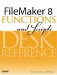# RGB()

Category: Text Formatting

 Syntax: RGB ( red; green; blue )

Parameters:

redAny number or numeric expression containing a value ranging from 0 to 255.

greenAny number or numeric expression containing a value ranging from 0 to 255.

blueAny number or numeric expression containing a value ranging from 0 to 255.

Data type returned: Number

Description:

Returns a number that represents a color.

To calculate this integer, the red, green, and blue values are combined using the following formula:

``` (red * 2562) + (green * 256) + blue
```

Use the RGB() function in conjunction with the TextColor() function to format text.

If a number above 255 is supplied as the parameter, the formula in the example still computes a result. If the result of the formula returns a value above the expected 0 to 16777215 range, the Mod (result; 16777216) is used to map the result into the expected range. So RGB (255; 255; 256), which returns a value one higher than white, returns the color black, just as 0 does.

Examples:

Table 6.1 lists the RGB values of some common colors.

##### Table 6.1. RGB Values

Function

Integer Result

Color

RGB (255; 0; 0)

16711680

Red

RGB (0; 255; 0)

65280

Green

RGB (0; 0; 255)

255

Blue

RGB (255; 255; 255)

16777215

White

RGB (0; 0; 0)

0

Black

RGB (24; 162; 75)

1614411

Dark Green

RGB (7; 13; 78)

462158

Dark Purple

RGB (23; 100; 148)

1533076

Bright BlueFileMaker 8 Functions and Scripts Desk Reference
ISBN: 0789735113
EAN: 2147483647
Year: 2004
Pages: 352